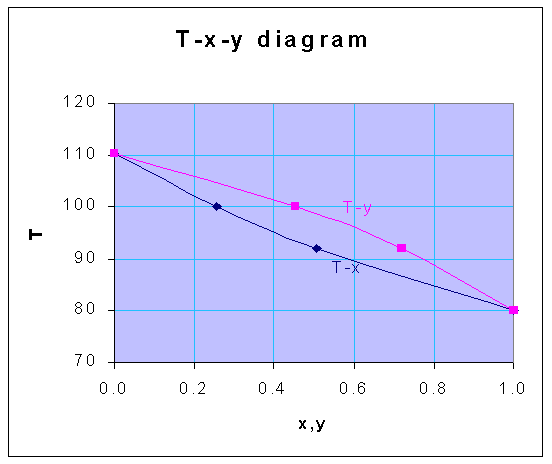### T-x-y Diagram of Ideal Solution

From the following vapor pressure data, construct the temperature - composition diagram at 1 atm, for the system benzene-toluene, assuming ideal solution behavior.

 Temperature oC Vapor pressure, mm Hg Benzene Toluene 80 760 300 92 1078 432 100 1344 559 110.4 1748 760

Calculations:

Mole fraction in liquid phase (x) is given by,

x = (pt - pB)/(pA - pB)

The corresponding equilibrium mole fraction in vapor phase (y) is,

y = pAx/pt

Using these equations, for the various temperatures given, the following data for x and y are obtained, for the total pressure of 1 atm

 T x y 80 1.000 1.000 92 0.508 0.720 100 0.256 0.453 110.4 0.000 0.000

From the above-calculated data, the following T-x-y diagram is drawn.HOME